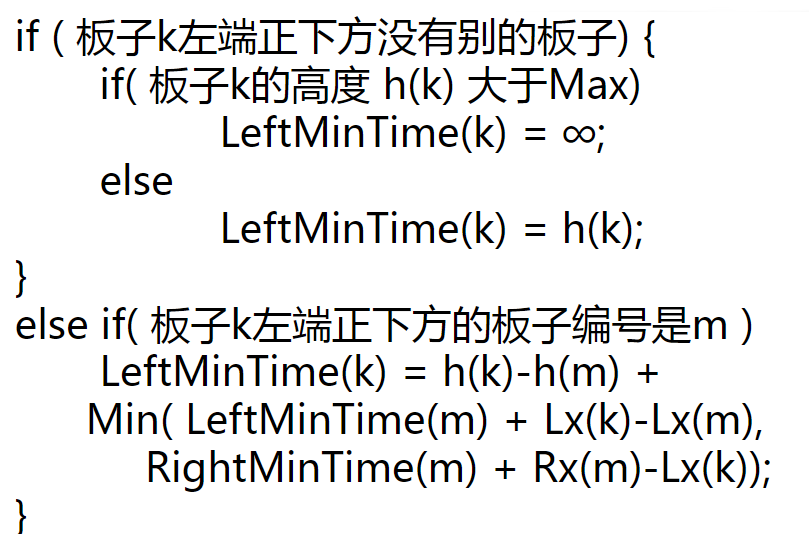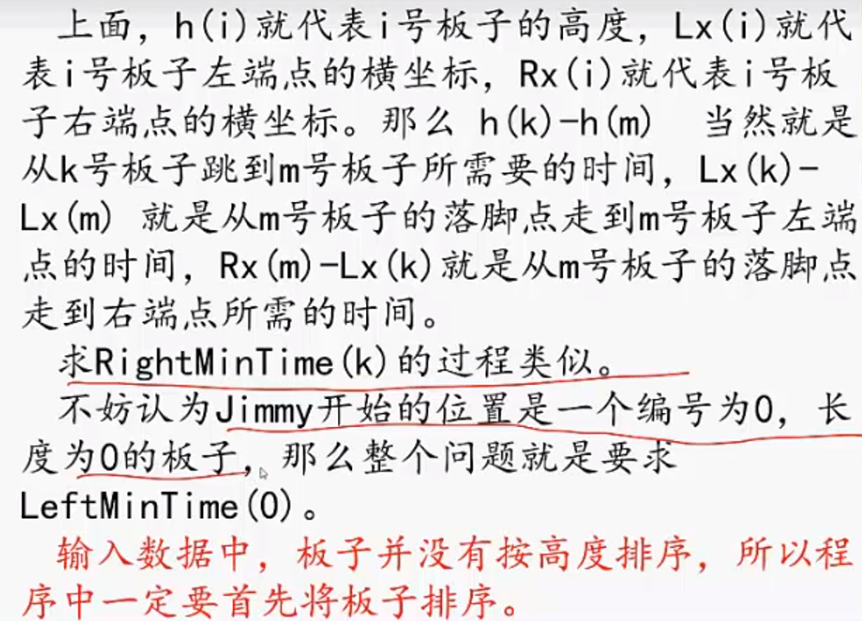# Help Jimmy (POJ 1661)

"Help Jimmy" 是在下图所示的场景上完成的游戏。Jimmy老鼠在时刻0从高于所有平台的某处开始下落，它的下落速度始终为1米/秒。当Jimmy落到某个平台上时，游戏者选择让它向左还是向右跑，它跑动的速度也是1米/秒。当Jimmy跑到平台的边缘时，开始继续下落。Jimmy每次下落的高度不能超过MAX米，不然就会摔死，游戏也会结束。

Input

Jimmy的大小和平台的厚度均忽略不计。如果Jimmy恰好落在某个平台的边缘，被视为落在平台上。所有的平台均不重叠或相连。测试数据保证问题一定有解。
Output

Sample Input
1
3 8 17 20
0 10 8
0 10 13
4 14 3
Sample Output
23#include<bits/stdc++.h>
const int N = 1003;
const int M = 20004;
const int INF = 0x3f3f3f;
using namespace std;
struct Time {
int x1, x2, h;
}a[N];
int dp[N];//0表示第i的木板左边到底的最短时间，1表示右边的时间
//这个dp其实就是问题中所需要求的时间
int max_h, n;
int cmp(Time c, Time b) {
return c.h> b.h;//从大到小排列
}
void LeftMinTime(int i) {
//往左
int k = i + 1;//k是i木板的下一个，整体是从下往上推的
while (k <= n && a[i].h - a[k].h <= max_h) {
if (a[i].x1 >= a[k].x1 && a[i].x1 <= a[k].x2) {
//上面这个是上面的木板可以往左跳到下面木板的条件
dp[i] = a[i].h - a[k].h + min(dp[k] + a[i].x1 - a[k].x1, dp[k] + a[k].x2 - a[i].x1);
//往左走是的时间是由两块板之间的距离，加上下一块板往左走或者往右走的最小值
return;
}
k++;
}
if (a[i].h - a[k].h > max_h) {
//不可以到达下一个平台
dp[i] = INF;
}
else {
//直接落地
dp[i] = a[i].h;
}
return;
}
void RightMinTime(int i) {
//往右
int k = i + 1;//k是i木板的下一个，整体是从下往上推的
while (k <= n && a[i].h - a[k].h <= max_h) {
if (a[i].x2 >= a[k].x1 && a[i].x2 <= a[k].x2) {
//上面这个是上面的木板可以往左跳到下面木板的条件
dp[i] = a[i].h - a[k].h + min(dp[k] + a[i].x2 - a[k].x1, dp[k] + a[k].x2 - a[i].x2);
//往左走是的时间是由两块板之间的距离，加上下一块板往左走或者往右走的最小值
return;
}
k++;
}
if (a[i].h - a[k].h > max_h) {
//不可以到达下一个平台
dp[i] = INF;
}
else {
//直接落地
dp[i] = a[i].h;
}
return;
}
int main() {
int t, x, y, i;
cin >> t;
while (t--) {
cin >> n >> x >> y >> max_h;
//后面是边界的设置，注意这个边界特征就是地面。
a.x1 = -20000;
a.x2 = 20000;
//地面的边界
a.h = 0;
//上面是地面，地面在第一层
a.x1 = a.x2 = x;
a.h = y;
//但是我有一个问题，
for (i = 2; i <= n + 1; i++) {
cin >> a[i].x1 >> a[i].x2 >> a[i].h;
}
sort(a, a + n + 2, cmp);
memset(dp, 0, sizeof(dp));
//memset这个函数还是要学会用的的确很方便
for (i = n; i >= 0; i--) {
//还有这个是从头开始的
LeftMinTime(i);
RightMinTime(i);
}
int time = min(dp, dp);
//很明显是从下到上递归
cout << time << endl;
return 0;
}
}

### 推荐这些文章：

【YBTOJ】【POJ 1821】Fence

\[f_{i,j}=\max(f_{i-1,j},f_{i,j-1},\max_{j-L_i\leq k \leq S_i-1}\{f...

A

B
C
D
E
F
G
H

(虽然贴代码也挺好但就是想试试新装的插件）
#include <iostream>
#include <cstring>
#include <algorithm>
#include <queue>
#include <math.h>
using namespace std;
const int N = 5e4 + 10;
int h[N], e[N], ne[N], idx;
int n, m, r;
bool st[N]; // 避免一个炸弹被多次引爆
struct Node
{
int x,...

#include<iostream>
using namespace std;
const int N = 1e5+10;
int n,m;
int s[N],num[N];
int main()
{
cin >> n >> m;
for(int i = 1; i <= n; i ++ )
{
cin >> num[i];
s[i] = s[i - 1] + num[i];
}
while(m -- )
{
int l,r...

code
#include<iostream>
#include<algorithm>
#include<complex>
#include<cstring>
using namespace std;
const int N=10000;
double a[N],b[N],c[N],d[N],e[N],f[N],t[N];//array t mean fly time
int main(){
ios::sync_with_stdio(false);
int n=0;
cin>>n;
for(int i=0;i<n;i+...

MySQL: = := @ @@ <=>

...

<P>&nbsp;</P>

...

poj3230Travel(dp动态规划)

1 #include<iostream>
2 #include<algorithm>
3 #include<cmath>
4 #include<cstring>
5 #include<cstdio>
6 #define inf 0x3f3f3f3f
7 using namespace std;
8 const int N = 105;
9
10 int dp[N][N], in[N][N], to[N][N];
11
12 int mai...

《九日集训》第十五轮 (第八讲) 二级指针

int **myMalloc(int r, int c, int* returnSize, int** returnColumnSizes) {
int i;
int **ret = (int **)malloc( sizeof(int *) * r ); // (1)
*returnColumnSizes = (int *)malloc( sizeof(int) * r ); // (2)
*returnSize = r; // (3)
f...

...

Maven <build>

1. <build>

1.1 <resources>

1.2 <plugins>

Java 1.8 编译

Spring 打包

2. <properties>

1. <build>
1.1 <resources>

<resource>
<directory>src/main/java</dire...

THE END